Search IntMath
Close

450+ Math Lessons written by Math Professors and Teachers

5 Million+ Students Helped Each Year

1200+ Articles Written by Math Educators and Enthusiasts

Simplifying and Teaching Math for Over 23 Years

# Is cube root the same as raising to power 1/3?

By Murray Bourne, 29 Oct 2015

I recently came across an interesting discrepancy involving the cube root function.

### Cube root

In Wolfram|Alpha (which uses the Mathematica computer algebra system at its backend), if you ask it to graph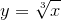, you get the following, as expected: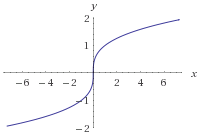[Image source: Wolfram|Alpha]

In the search box, I put "cube root of x", and it stated the "Result" was correctly written as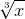.

This graph is the reflection of the graph y = x3 in the line y = x. They are inverse functions.

We know that that cube root of a negative number is negative, so for example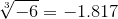, and we can see this makes sense on the graph above.

Wolfram|Alpha states there is one root (x = 0), and the domain and range are all real numbers, which is consistent with the graph above.

NOTE: In tiny font, Wolfram|Alpha states:

Assuming "cube root of" is the real-valued root.

There is an option to see the "principal root", but this just gave the same result.

### Raising to the power 1/3

We learn early in the study of roots and fractional powers that we can write roots in terms of fractional exponents. In general, that means: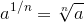So for square root we have: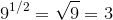and for cube root: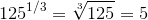.

So we would expect the graph for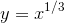to be the same as the one for.

But it isn't. Here's what Wolfram|Alpha returns when I ask it to graph: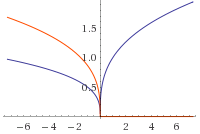[Image source: Wolfram|Alpha]

The blue curve is labeled "real part" and the red curve is "imaginary part".

Curiously, the "Input" value is stated as:, but that's not actually what I entered. So part of the answer regards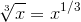, but the rest of the answer does not.

We know from the section on complex roots (see especially Exercise 4 at the end), that a cubic equation will have 3 roots (just as a quadratic equation has 2 roots). These 3 roots may be all real, or a mix of real and complex roots.

Wolfram|Alpha is right to indicate there are imaginary parts, but is their graph correct? Surely the cube root of a negative number should be negative?### Example: What are all the cube roots of −8?

I zoomed out a bit to get this graph, and added some guide segments (in green):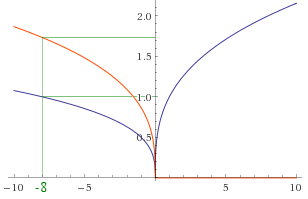[Image source: Wolfram|Alpha]

Using the same thinking as Exercise 4 mentioned earlier, the complex solutions for x3 = −8 should be 120° apart, giving (where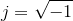):

x = −2

x = 1 + 1.73j

x = 1 − 1.73j

The above graph does give us one of these solutions (the middle one, since we can see the real part is 1 and the imaginary part is 1.73), but it doesn't give the other two solutions.

Once again, the page tells us it is assuming the "principal root", and gives us the option to choose the "real-valued root". If we do that this time, we get the real root only version, looking like the graph at the top of the page.

Scientific Notebook gives the following 2 graphs, which I've overlaid.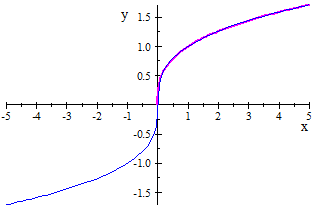The blue graph is, and Scientific Notebook gives the full real solution (in first and third quadrants), while the magenta (pink) graph,is in the positive quadrant only.

Both Geogebra and Desmos give the same "full real value" graph for bothand.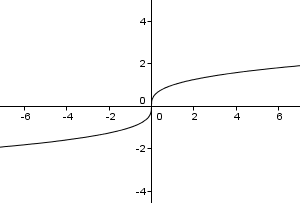## Similar issue to square root

I've written before about the number of solutions for √16. The answer there is one solution of course, whereas if you are asked to solve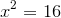, you will get 2 solutions.

Wolfram|Alpha and Scientific Notebook recognise there is a difference between(there is one "principal" answer each time) and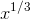, where we need to remember the complex roots.

## Conclusion

Don't just accept the computer's word for it when it gives you a graph, or the solution for some equation. It's doing its best to figure out what you want to know, but can't be expected to know the full context of your query, or necessarily give you all the possible answers.

I find it strange that Wolfram|Alpha gives only a partial graphical answer for the cube root of −8. The user has to do quite a bit of thinking to extract the complete answer from it.### 6 Comments on “Is cube root the same as raising to power 1/3?”

1. cccccttttt says:

Part of problem is fuzzy statement of domain and range
along with the rule.

Is it Real to Real Real to Complex Complex to Real or
Complex to Complex?

Students should also take away that math teachers sometimes
are the cause of these situations.

(-:

2. Tommy Peter says:

cube root of a number is just the same as raising to the power 1/3. for example if you take cube root of a number or if you raise that same number to power 1/3, you will still come up with the same numerical value.
proof. cube root of 8 is 2 and also 8 raise to power 1/3 is 2.so I strongly believe that cube root of a number is another way of raising this same number to power 1/3.

3. But.. says:

Cube root of -1 is the same as (-1)^(1/3) which is the same as (-1)^(2/6). Cube root of -1 equals -1 but (-1)^(2/6) equals 1. Altough they seem to be the same they give different answers.

4. Murray says:

@Tommy: Did you read the article?

@But: There are contradictions in your statement, since you say they are the same, then not the same since they give different answers!

5. Zeltrax247 says:

cube root of a negative number is not negative, it is just one of the three cube roots but not the principal root.

A principal root is defined to be the complex root that has the least argument among all roots. For eg: out of the three cube roots of -8, 1+i.sqrt(3) has the least argument (pi/3) and hence is the principal root, not -2.

So, wolfram or any other website would be correct if they returned "1+i.sqrt(3)" when queried for "cuberoot(-8)", not if they returned -2.

And finally, there is absolutely no difference between cuberoot, ∛ and ^(1/3). The latter two are just symbolic representations of the more lingual "cuberoot(something)"; nothing more, nothing less!

https://en.wikipedia.org/wiki/Nth_root

6. Jacob Johnson says:

Just because you can give an example doesn't mean it's always right.
(Euler's conjecture, all numbers are prime (2, 4, 6, 8, etc.!)

### Comment Preview

HTML: You can use simple tags like <b>, <a href="...">, etc.

To enter math, you can can either:

1. Use simple calculator-like input in the following format (surround your math in backticks, or qq on tablet or phone):
a^2 = sqrt(b^2 + c^2)
(See more on ASCIIMath syntax); or
2. Use simple LaTeX in the following format. Surround your math with $$ and $$.
$$\int g dx = \sqrt{\frac{a}{b}}$$
(This is standard simple LaTeX.)

NOTE: You can mix both types of math entry in your comment.